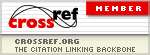It is the cache of http://www.ccsenet.org/journal/index.php/ijsp/article/view/23491. It is a snapshot of the page. The current page could have changed in the meantime.
Tip: To quickly find your search term on this page, press Ctrl+F or ⌘-F (Mac) and use the find bar.

The McDonald Generalized Beta-Binomial Distribution: A New Binomial Mixture Distribution and Simulation Based Comparison with Its Nested Distributions in Handling Overdispersion | Manoj | International Journal of Statistics and Probability

### The McDonald Generalized Beta-Binomial Distribution: A New Binomial Mixture Distribution and Simulation Based Comparison with Its Nested Distributions in Handling Overdispersion

Chandrabose Manoj, Pushpa Wijekoon, Roshan Darshana Yapa

#### Abstract

The binomial outcome data are widely encountered in many real world applications. The Binomial distribution often fails to model the binomial outcomes since the variance of the observed binomial outcome data exceeds the nominal Binomial distribution variance, a phenomenon known as overdispersion. One way of handling overdispersion is modeling the success probability of the Binomial distribution using a continuous distribution defined on the standard unit interval. The resultant general class of univariate discrete distributions is known as the class of Binomial mixture distributions. The Beta-Binomial (BB) distribution is a prominent member of this class of distributions. The Kumaraswamy-Binomial (KB) distribution is another recent member of this class. In this paper we focus the emphasis on the McDonald's Generalized Beta distribution of the first kind as the mixing distribution and introduce a new Binomial mixture distribution called the McDonald Generalized Beta-Binomial distribution(McGBB). Some theoretical properties of McGBB are discussed. The parameters of the McGBB distribution are estimated via maximum likelihood estimation technique. A real world dataset is modeled by using the new McGBB mixture distribution, and it is shown that this model gives better fit than its nested models. Finally, an extended simulation study is presented to compare the McGBB distribution with its nested distributions in handling overdispersed binomial outcome data.

Full Text: PDF DOI: 10.5539/ijsp.v2n2p24

### Refbacks

• There are currently no refbacks.